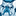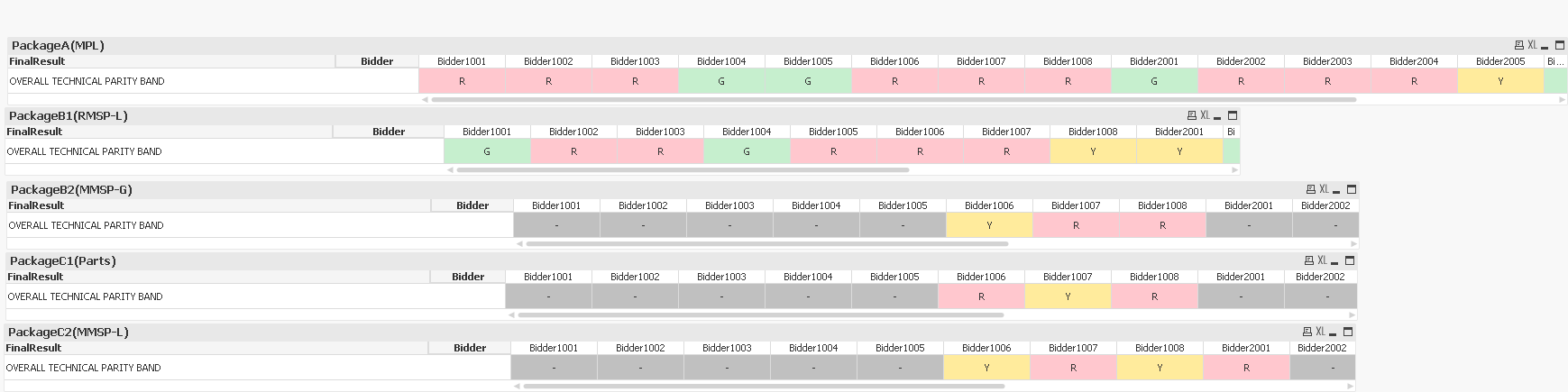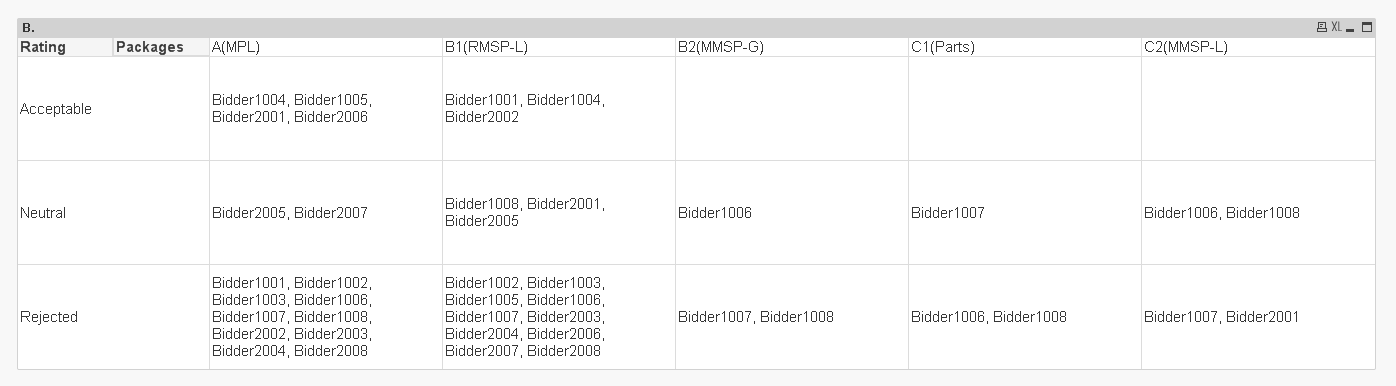# QlikView App Dev

Discussion Board for collaboration related to QlikView App Development.

cancel
Showing results for
Did you mean:Creator III

## Help with Expression for Pivot table to get output of multiple nested if conditions

Hello,

I have a 5 separate Pivot tables which give the results of a bidder rating(G='Acceptable',Y='Neutral',R='Rejected) based on multiple conditions for 5 Packages as shown in the image below:Now I want to create a summary table of the above 5 tables, giving the list of Bidders falling into each rating bucket. Something like this:

RatingA(MPL)B1(RMSP-L)B2(MMSP-G)C1(Parts)C2(MMSP-L)
AcceptableBidder1004,Bidder1005,Bidder2001,Bidder2006Bidder1001,Bidder1004,Bidder2002
NeutralBidder2005,Bidder2007Bidder1008,Bidder2001,Bidder2005
Rejected

Bidder1001,Bidder1002,Bidder1003,Bidder1006,Bidder1007,Bidder1008,

Bidder2002,Bidder2003,Bidder2004,Bidder2008

Bidder1002,Bidder1003,Bidder1005,Bidder1006,Bidder1007,Bidder2003,

Bidder2004,Bidder2006,Bidder2007,Bidder2008

Please kindly help me with getting the expression right for this summary table. I have tried the following expression:

Pick(Match(Rating,'Acceptable','Neutral','Rejected'),

if(if(FinalResult='OVERALL TECHNICAL PARITY BAND' and Wildmatch(Bidder,'Bidder1***')=1 and (Count({<Appendix={'Appendix V'},No={'1A'},A={'COMPLY'}>}Items)=0) or (Count({<Appendix={'Appendix V'},={'COMPLY'}>}distinct No)<>Count({<Appendix={'Appendix V'},-={'N/A'}>}distinct No)) or (Package='Package A' and [HSE Evaluation Result]='R') or (Sum(If(VC.F22 ...

(Flag9='Fail' or Flag10='Fail' or Flag11='Fail' or Flag12='Fail' or Flag13='Fail' or Flag14='Fail') or (Flag15='Fail' or Flag16='Fail' or Flag17='Fail' or Flag18='Fail' or Flag19='Fail' or Flag20='Fail') or (Count({<Appendix={'Appendix V'},No={'14'},={'COMPLY'}>}distinct No)=0) or

(Package='Package A' and [Financial Evaluaton Result]='R'),'R',

if(FinalResult='OVERALL TECHNICAL PARITY BAND' and Wildmatch(Bidder,'Bidder1***')=1 and (Count({<Appendix={'Appendix V'},No={'1A'},A={'COMPLY'}>}Items)=1) and (Count({<Appendix={'Appendix V'},={'COMPLY'}>}distinct No)=Count({<Appendix={'Appendix V'},-={'N/A'}>}distinct No)) and (Sum(If(VC.F22 = 'CV Submitted by Bidder', Responses0))>=4) and

(Count({<Appendix={'Appendix V'},No={'14'},={'COMPLY'}>}distinct No)=1) and (Package='Package A' and [HSE Evaluation Result]='Y') or (Flag12='Y...

'Y',

if(FinalResult='OVERALL TECHNICAL PARITY BAND' and Wildmatch(Bidder,'Bidder2***')=1 and (Count({<Appendix={'Appendix V'},No={'1A'},A={'COMPLY'}>}Items)=0) or (Sum({<Appendix={'Appendix V(D) Time'}>})<60 and Sum({<Appendix={'Appendix V(D) Value'}>})<3000000) or (Count({<Appendix={'Appendix V'},={'COMPLY'}>}distinct No)<>Count({<Appendix={'Appendix V'},-={'N/A'}>}distinct No)) or (Package='Package A' and [HSE Evaluation Result]='R') or (Sum(If(VC.F22 ...

(Flag9='Fail' or Flag10='Fail' or Flag11='Fail' or Flag12='Fail' or Flag13='Fail' or Flag14='Fail') or (Flag15='Fail' or Flag16='Fail' or Flag17='Fail' or Flag18='Fail' or Flag19='Fail' or Flag20='Fail') or (Count({<Appendix={'Appendix V'},No={'14'},={'COMPLY'}>}distinct No)=0) or

(Package='Package A' and [Financial Evaluaton Result]='R'),'R',

if(FinalResult='OVERALL TECHNICAL PARITY BAND' and Wildmatch(Bidder,'Bidder2***')=1 and (Count({<Appendix={'Appendix V'},No={'1A'},A={'COMPLY'}>}Items)=1) and (Sum({<Appendix={'Appendix V(D) Time'}>})>=60 or Sum({<Appendix={'Appendix V(D) Value'}>})>=3000000) and (Count({<Appendix={'Appendix V'},={'COMPLY'}>}distinct No)=Count({<Appendix={'Appendix V'},-={'N/A'}>}distinct No)) and (Sum(If(VC.F22 = 'CV Submitted by Bidder', Responses0))>=4) and

(Count({<Appendix={'Appendix V'},No={'14'},={'COMPLY'}>}distinct No)=1) and (Flag12='Yellow') or (Flag18='Yellow') or (Package='Package A' and ...

'Y','G'))))='G',Bidder))

where the multiple nested if conditions give the criteria for rating the bidder but it seems it's not correct as the table becomes blank.. I'm attaching the QV file to the post. You can find the summary table in the tab named 'Summary'. Thanks in advance!

1 Solution

Accepted SolutionsMVP

This5 RepliesMVP

ThisCreator III
Author

Wow. Excellent! You put the pick match expression in a variable and then used another pick match function in the actual table with Concat(Aggr). Can you please explain what the Concat(Aggr is helping us accomplish in this expression? Thanks a ton again Sunny!MVP

Concatenating multiple Bidders into a single cellCreator III
Author

Got it! So I guess when you have such long nested if formulae and need to use their output in another expression the best way would be to go with variables. Lesson learnt! Thanks a lot Sunny! Really really appreciate it!MVP

Yes, if I had to repeat the same expression three times, how horrible was it going to look... to make it look small (and deceive you) I used variable....